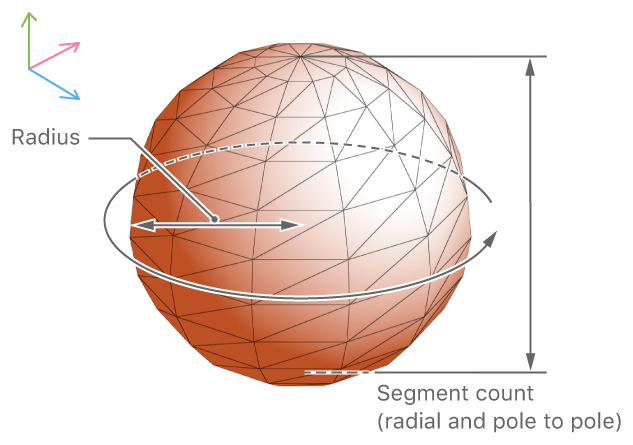Class

SCNSphere

A sphere (or ball or globe) geometry.

OverviewFigure 1 A sphere and its properties

A sphere defines a surface whose every point is equidistant from its center, which is placed at the origin of its local coordinate space. You define the size of the sphere in all three dimensions using its radius property.

SceneKit approximates the curved surface of a sphere using a mesh of polygons. There are two options for constructing the mesh:

• By default, SceneKit constructs the sphere using a rectangular grid, like the lines of latitude and longitude on a globe of the Earth. The sphere has a vertex at each pole, and its segmentCount property determines both the number of divisions along its surface from one pole to the other (or lines of latitude) and the number of divisions around its circumference in a horizontal plane (or lines of longitude).

• If you set the sphere’s isGeodesic property to true, SceneKit constructs the sphere by successively subdividing the triangular surfaces of a regular icosahedron. For a geodesic sphere, the SCNSphere property scales logarithmically to determine the number of subdivisions, roughly approximating the number of vertices generated by a non-geodesic sphere of the same segment count.

With either approximation, increasing the SCNSphere property produces more vertices and a more smoothly curved surface, which can improve rendering quality at a cost to rendering performance.

To position and orient a sphere in a scene, attach it to the geometry property of an SCNNode object.

Topics

Creating a Sphere

Creates a sphere geometry with the specified radius.

Adjusting a Sphere’s Dimensions

The radius of the sphere. Animatable.

var isGeodesic: Bool

A Boolean value specifying whether SceneKit uses a geodesic polygon mesh to render the sphere.

var segmentCount: Int

A number determining the detail of the polygon mesh SceneKit uses to render the sphere. Animatable.

Relationships

Basic Shapes

class SCNFloor

A plane that can optionally display a reflection of the scene above it.

class SCNBox

A six-sided polyhedron geometry whose faces are all rectangles, optionally with rounded edges and corners.

class SCNCapsule

A right circular cylinder geometry whose ends are capped with hemispheres.

class SCNCone

A right circular cone or frustum geometry.

class SCNCylinder

A right circular cylinder geometry.

class SCNPlane

A rectangular, one-sided plane geometry of specified width and height.

class SCNPyramid

A right rectangular pyramid geometry.

class SCNTorus

A torus, or ring-shaped geometry.

class SCNTube

A tube or pipe geometry—a right circular cylinder with a circular hole along its central axis.